# Data mining approaches and methods

5 Aug 2018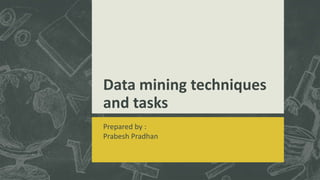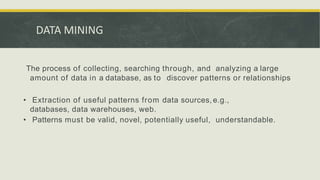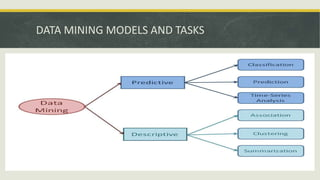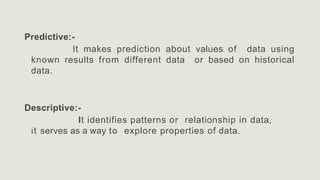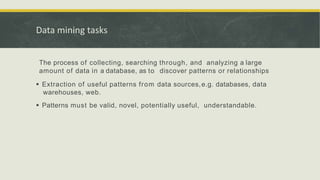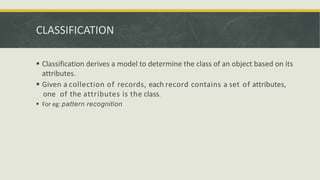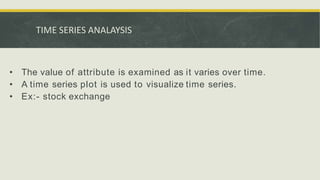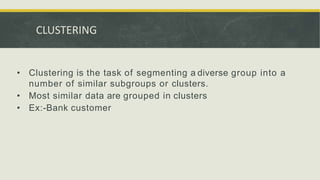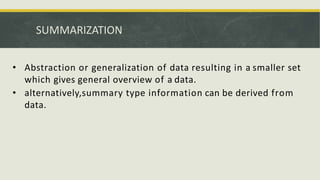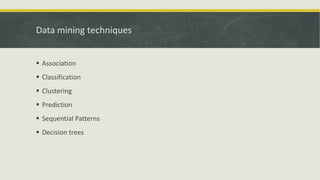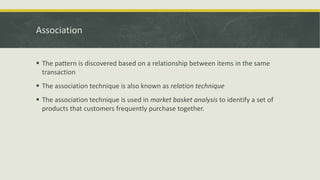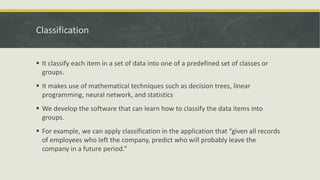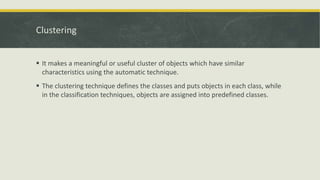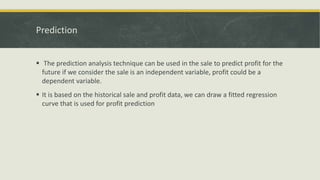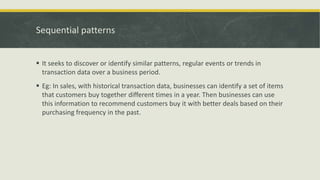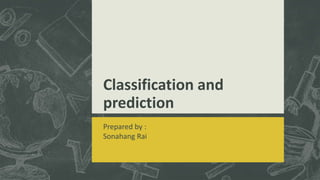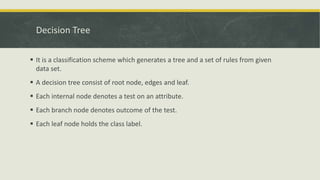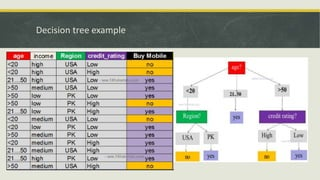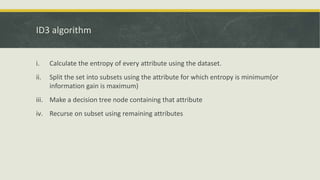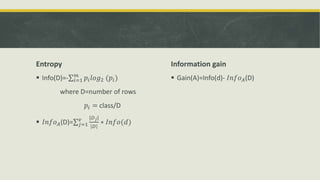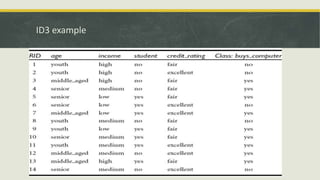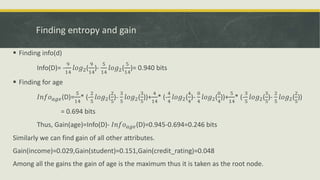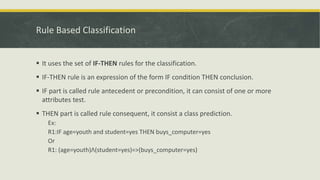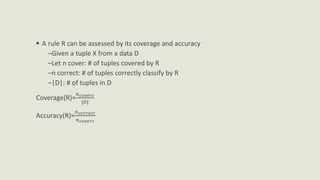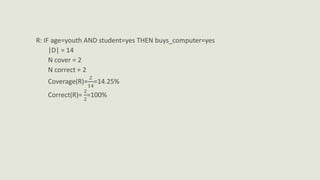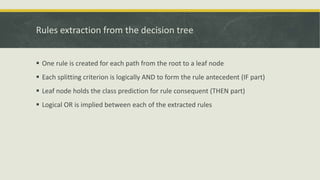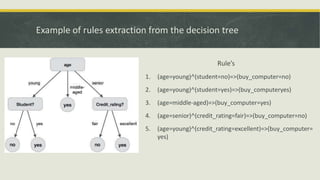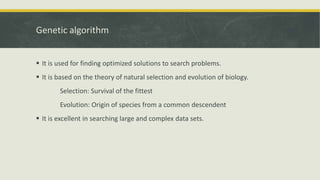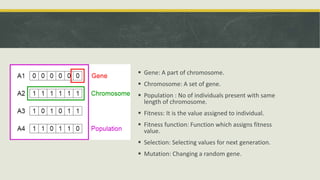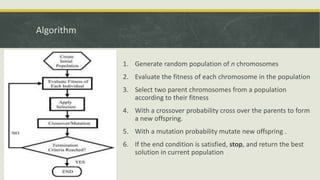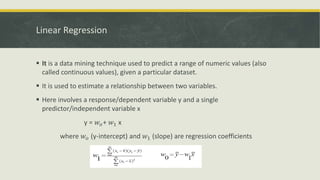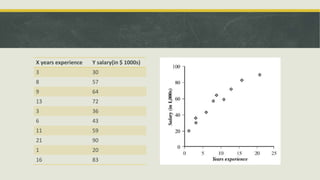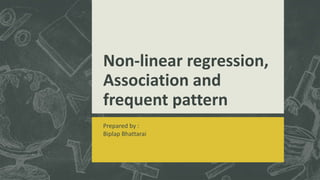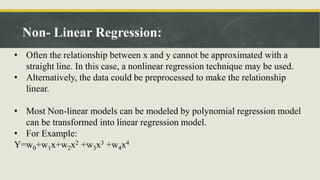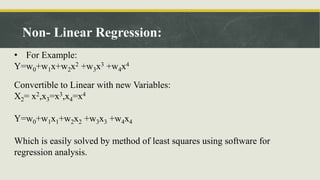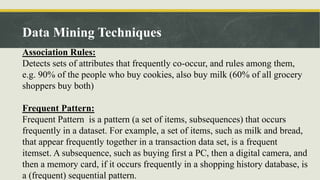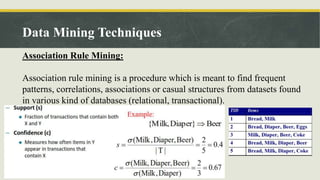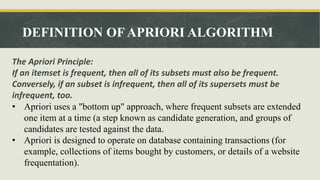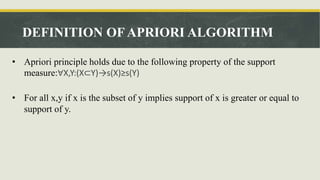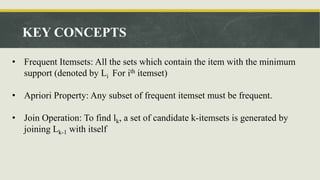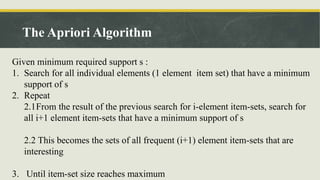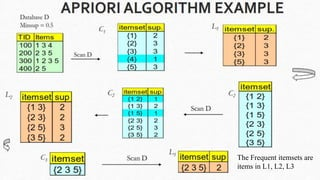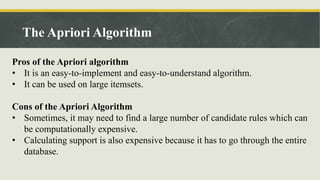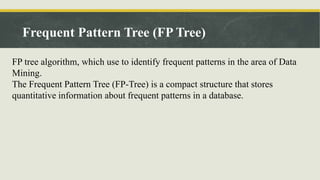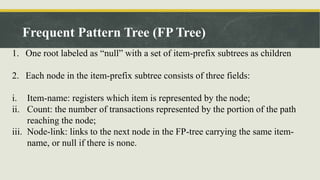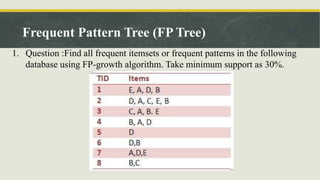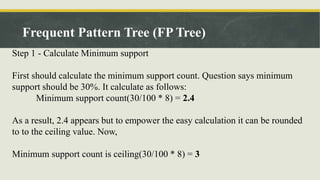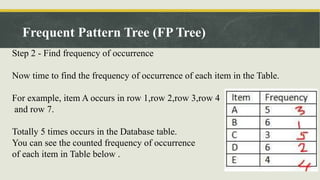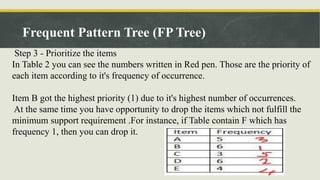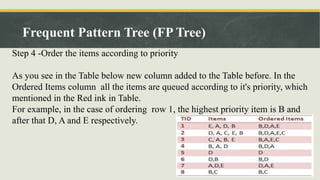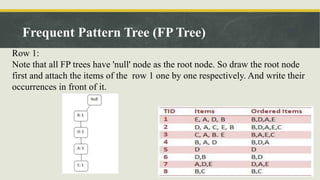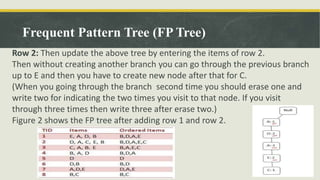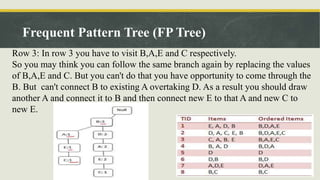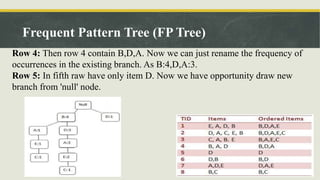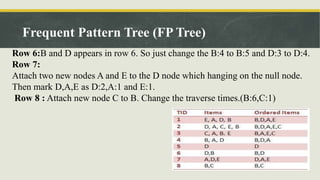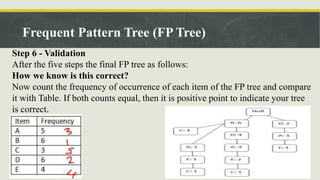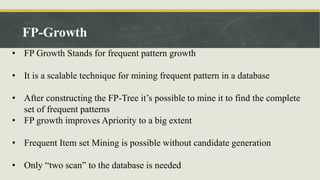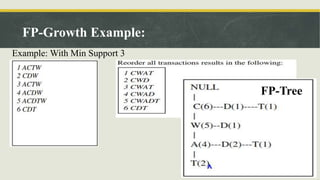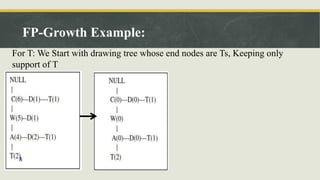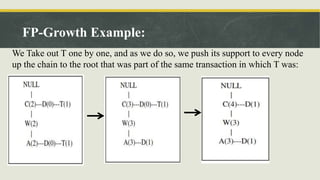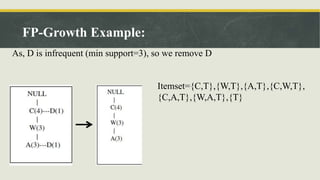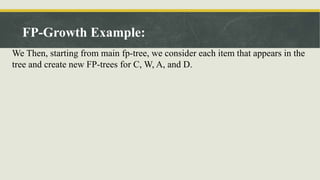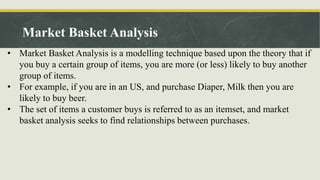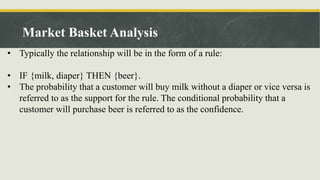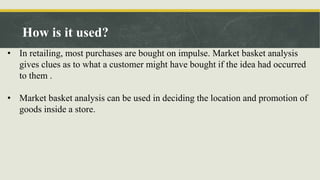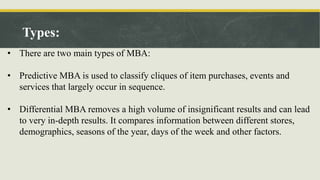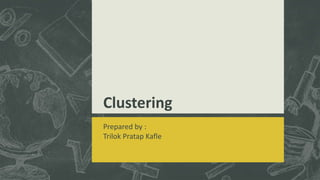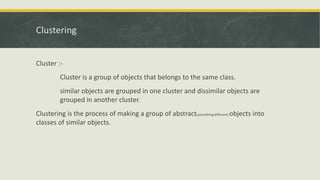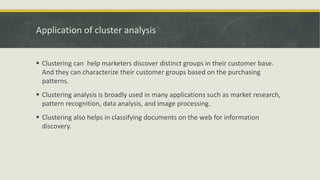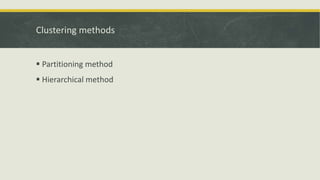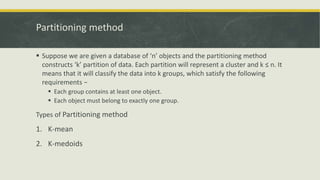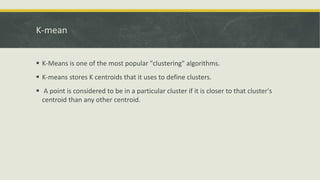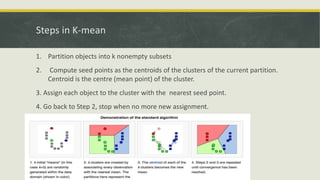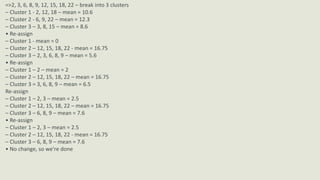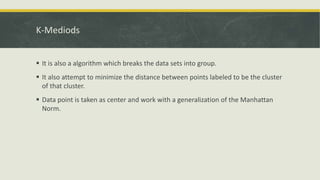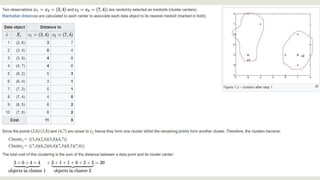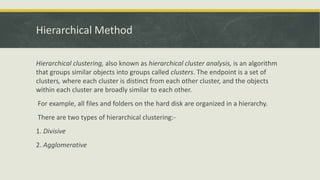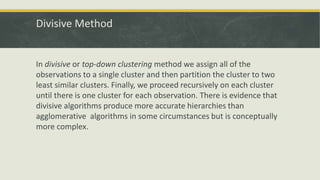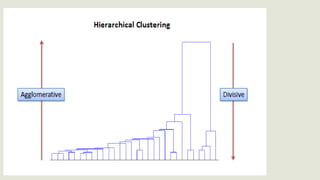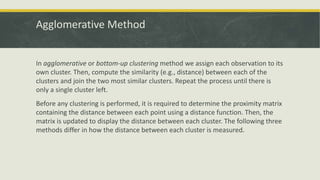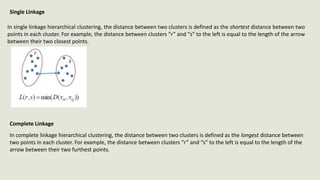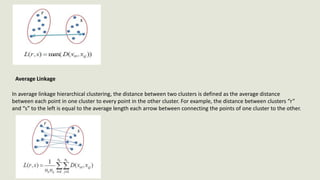1 sur 85

### Data mining approaches and methods

• 1. Data mining techniques and tasks Prepared by : Prabesh Pradhan
• 2. The process of collecting, searching through, and analyzing a large amount of data in a database, as to discover patterns or relationships • Extraction of useful patterns from data sources, e.g., databases, data warehouses, web. • Patterns must be valid, novel, potentially useful, understandable. DATA MINING
• 3. DATA MINING MODELS AND TASKS
• 4. Predictive:- It makes prediction about values of data using known results from different data or based on historical data. Descriptive:- It identifies patterns or relationship in data, it serves as a way to explore properties of data.
• 5. Data mining tasks The process of collecting, searching through, and analyzing a large amount of data in a database, as to discover patterns or relationships  Extraction of useful patterns from data sources,e.g. databases, data warehouses, web.  Patterns must be valid, novel, potentially useful, understandable.
• 6. CLASSIFICATION  Classification derives a model to determine the class of an object based on its attributes.  Given a collection of records, each record contains a set of attributes, one of the attributes is the class.  For eg: pattern recognition
• 7. • The value of attribute is examined as it varies over time. • A time series plot is used to visualize time series. • Ex:- stock exchange TIME SERIES ANALAYSIS
• 8. • Clustering is the task of segmenting a diverse group into a number of similar subgroups or clusters. • Most similar data are grouped in clusters • Ex:-Bank customer CLUSTERING
• 9. • Abstraction or generalization of data resulting in a smaller set which gives general overview of a data. • alternatively,summary type information can be derived from data. SUMMARIZATION
• 10. Data mining techniques  Association  Classification  Clustering  Prediction  Sequential Patterns  Decision trees
• 11. Association  The pattern is discovered based on a relationship between items in the same transaction  The association technique is also known as relation technique  The association technique is used in market basket analysis to identify a set of products that customers frequently purchase together.
• 12. Classification  It classify each item in a set of data into one of a predefined set of classes or groups.  It makes use of mathematical techniques such as decision trees, linear programming, neural network, and statistics  We develop the software that can learn how to classify the data items into groups.  For example, we can apply classification in the application that “given all records of employees who left the company, predict who will probably leave the company in a future period.”
• 13. Clustering  It makes a meaningful or useful cluster of objects which have similar characteristics using the automatic technique.  The clustering technique defines the classes and puts objects in each class, while in the classification techniques, objects are assigned into predefined classes.
• 14. Prediction  The prediction analysis technique can be used in the sale to predict profit for the future if we consider the sale is an independent variable, profit could be a dependent variable.  It is based on the historical sale and profit data, we can draw a fitted regression curve that is used for profit prediction
• 15. Sequential patterns  It seeks to discover or identify similar patterns, regular events or trends in transaction data over a business period.  Eg: In sales, with historical transaction data, businesses can identify a set of items that customers buy together different times in a year. Then businesses can use this information to recommend customers buy it with better deals based on their purchasing frequency in the past.
• 16. Classification and prediction Prepared by : Sonahang Rai
• 17. Decision Tree  It is a classification scheme which generates a tree and a set of rules from given data set.  A decision tree consist of root node, edges and leaf.  Each internal node denotes a test on an attribute.  Each branch node denotes outcome of the test.  Each leaf node holds the class label.
• 18. Decision tree example
• 19. ID3 algorithm i. Calculate the entropy of every attribute using the dataset. ii. Split the set into subsets using the attribute for which entropy is minimum(or information gain is maximum) iii. Make a decision tree node containing that attribute iv. Recurse on subset using remaining attributes
• 20. Entropy  Info(D)=- 𝑖=1 𝑚 𝑝𝑖 𝑙𝑜𝑔2 (𝑝𝑖) where D=number of rows 𝑝𝑖 = class/D  𝐼𝑛𝑓𝑜 𝐴(D)= 𝑗=1 𝑣 |𝐷 𝑗| 𝐷 ∗ 𝐼𝑛𝑓𝑜(𝑑) Information gain  Gain(A)=Info(d)- 𝐼𝑛𝑓𝑜 𝐴(D)
• 21. ID3 example
• 22. Finding entropy and gain  Finding info(d) Info(D)= - 9 14 𝑙𝑜𝑔2( 9 14 )- 5 14 𝑙𝑜𝑔2( 5 14 )= 0.940 bits  Finding for age 𝐼𝑛𝑓𝑜 𝑎𝑔𝑒(D)= 5 14 * (- 2 5 𝑙𝑜𝑔2( 2 5 )- 3 5 𝑙𝑜𝑔2( 3 5 ))+ 4 14 * (- 4 4 𝑙𝑜𝑔2( 4 4 )- 0 4 𝑙𝑜𝑔2( 0 4 ))+ 5 14 * (- 3 5 𝑙𝑜𝑔2( 3 5 )- 2 5 𝑙𝑜𝑔2( 2 5 )) = 0.694 bits Thus, Gain(age)=Info(D)- 𝐼𝑛𝑓𝑜 𝑎𝑔𝑒(D)=0.945-0.694=0.246 bits Similarly we can find gain of all other attributes. Gain(income)=0.029,Gain(student)=0.151,Gain(credit_rating)=0.048 Among all the gains the gain of age is the maximum thus it is taken as the root node.
• 23. Rule Based Classification  It uses the set of IF-THEN rules for the classification.  IF-THEN rule is an expression of the form IF condition THEN conclusion.  IF part is called rule antecedent or precondition, it can consist of one or more attributes test.  THEN part is called rule consequent, it consist a class prediction. Ex: R1:IF age=youth and student=yes THEN buys_computer=yes Or R1: (age=youth)Λ(student=yes)=>(buys_computer=yes)
• 24.  A rule R can be assessed by its coverage and accuracy –Given a tuple X from a data D –Let n cover: # of tuples covered by R –n correct: # of tuples correctly classify by R –|D|: # of tuples in D Coverage(R)= 𝑛 𝑐𝑜𝑣𝑒𝑟𝑠 |𝐷| Accuracy(R)= 𝑛 𝑐𝑜𝑟𝑟𝑒𝑐𝑡 𝑛 𝑐𝑜𝑣𝑒𝑟𝑠
• 25. R: IF age=youth AND student=yes THEN buys_computer=yes |D| = 14 N cover = 2 N correct = 2 Coverage(R)= 2 14 =14.25% Correct(R)= 2 2 =100%
• 26. Rules extraction from the decision tree  One rule is created for each path from the root to a leaf node  Each splitting criterion is logically AND to form the rule antecedent (IF part)  Leaf node holds the class prediction for rule consequent (THEN part)  Logical OR is implied between each of the extracted rules
• 28. Genetic algorithm  It is used for finding optimized solutions to search problems.  It is based on the theory of natural selection and evolution of biology. Selection: Survival of the fittest Evolution: Origin of species from a common descendent  It is excellent in searching large and complex data sets.
• 29.  Gene: A part of chromosome.  Chromosome: A set of gene.  Population : No of individuals present with same length of chromosome.  Fitness: It is the value assigned to individual.  Fitness function: Function which assigns fitness value.  Selection: Selecting values for next generation.  Mutation: Changing a random gene.
• 30. Algorithm 1. Generate random population of n chromosomes 2. Evaluate the fitness of each chromosome in the population 3. Select two parent chromosomes from a population according to their fitness 4. With a crossover probability cross over the parents to form a new offspring. 5. With a mutation probability mutate new offspring . 6. If the end condition is satisfied, stop, and return the best solution in current population
• 31. Linear Regression  It is a data mining technique used to predict a range of numeric values (also called continuous values), given a particular dataset.  It is used to estimate a relationship between two variables.  Here involves a response/dependent variable y and a single predictor/independent variable x y = 𝑤𝑜+ 𝑤1 x where 𝑤𝑜 (y-intercept) and 𝑤1 (slope) are regression coefficients
• 32. X years experience Y salary(in \$ 1000s) 3 30 8 57 9 64 13 72 3 36 6 43 11 59 21 90 1 20 16 83
• 33. Non-linear regression, Association and frequent pattern Prepared by : Biplap Bhattarai
• 34. • Often the relationship between x and y cannot be approximated with a straight line. In this case, a nonlinear regression technique may be used. • Alternatively, the data could be preprocessed to make the relationship linear. • Most Non-linear models can be modeled by polynomial regression model can be transformed into linear regression model. • For Example: Y=w0+w1x+w2x2 +w3x3 +w4x4 Non- Linear Regression:
• 35. • For Example: Y=w0+w1x+w2x2 +w3x3 +w4x4 Convertible to Linear with new Variables: X2= x2,x3=x3,x4=x4 Y=w0+w1x1+w2x2 +w3x3 +w4x4 Which is easily solved by method of least squares using software for regression analysis. Non- Linear Regression:
• 36. Association Rules: Detects sets of attributes that frequently co-occur, and rules among them, e.g. 90% of the people who buy cookies, also buy milk (60% of all grocery shoppers buy both) Frequent Pattern: Frequent Pattern is a pattern (a set of items, subsequences) that occurs frequently in a dataset. For example, a set of items, such as milk and bread, that appear frequently together in a transaction data set, is a frequent itemset. A subsequence, such as buying first a PC, then a digital camera, and then a memory card, if it occurs frequently in a shopping history database, is a (frequent) sequential pattern. Data Mining Techniques
• 37. Association Rule Mining: Association rule mining is a procedure which is meant to find frequent patterns, correlations, associations or casual structures from datasets found in various kind of databases (relational, transactional). Data Mining Techniques
• 38. The Apriori Principle: If an itemset is frequent, then all of its subsets must also be frequent. Conversely, if an subset is infrequent, then all of its supersets must be infrequent, too. • Apriori uses a "bottom up" approach, where frequent subsets are extended one item at a time (a step known as candidate generation, and groups of candidates are tested against the data. • Apriori is designed to operate on database containing transactions (for example, collections of items bought by customers, or details of a website frequentation). DEFINITION OF APRIORI ALGORITHM
• 39. • Apriori principle holds due to the following property of the support measure:∀X,Y:(X⊂Y)→s(X)≥s(Y) • For all x,y if x is the subset of y implies support of x is greater or equal to support of y. DEFINITION OF APRIORI ALGORITHM
• 40. • Frequent Itemsets: All the sets which contain the item with the minimum support (denoted by Li For ith itemset) • Apriori Property: Any subset of frequent itemset must be frequent. • Join Operation: To find lk, a set of candidate k-itemsets is generated by joining Lk-1 with itself KEY CONCEPTS
• 41. Given minimum required support s : 1. Search for all individual elements (1 element item set) that have a minimum support of s 2. Repeat 2.1From the result of the previous search for i-element item-sets, search for all i+1 element item-sets that have a minimum support of s 2.2 This becomes the sets of all frequent (i+1) element item-sets that are interesting 3. Until item-set size reaches maximum The Apriori Algorithm
• 42. The Frequent itemsets are items in L1, L2, L3
• 43. Pros of the Apriori algorithm • It is an easy-to-implement and easy-to-understand algorithm. • It can be used on large itemsets. Cons of the Apriori Algorithm • Sometimes, it may need to find a large number of candidate rules which can be computationally expensive. • Calculating support is also expensive because it has to go through the entire database. The Apriori Algorithm
• 44. FP tree algorithm, which use to identify frequent patterns in the area of Data Mining. The Frequent Pattern Tree (FP-Tree) is a compact structure that stores quantitative information about frequent patterns in a database. Frequent Pattern Tree (FP Tree)
• 45. 1. One root labeled as “null” with a set of item-prefix subtrees as children 2. Each node in the item-prefix subtree consists of three fields: i. Item-name: registers which item is represented by the node; ii. Count: the number of transactions represented by the portion of the path reaching the node; iii. Node-link: links to the next node in the FP-tree carrying the same item- name, or null if there is none. Frequent Pattern Tree (FP Tree)
• 46. 1. Question :Find all frequent itemsets or frequent patterns in the following database using FP-growth algorithm. Take minimum support as 30%. Frequent Pattern Tree (FP Tree)
• 47. Step 1 - Calculate Minimum support First should calculate the minimum support count. Question says minimum support should be 30%. It calculate as follows: Minimum support count(30/100 * 8) = 2.4 As a result, 2.4 appears but to empower the easy calculation it can be rounded to to the ceiling value. Now, Minimum support count is ceiling(30/100 * 8) = 3 Frequent Pattern Tree (FP Tree)
• 48. Step 2 - Find frequency of occurrence Now time to find the frequency of occurrence of each item in the Table. For example, item A occurs in row 1,row 2,row 3,row 4 and row 7. Totally 5 times occurs in the Database table. You can see the counted frequency of occurrence of each item in Table below . Frequent Pattern Tree (FP Tree)
• 49. Step 3 - Prioritize the items In Table 2 you can see the numbers written in Red pen. Those are the priority of each item according to it's frequency of occurrence. Item B got the highest priority (1) due to it's highest number of occurrences. At the same time you have opportunity to drop the items which not fulfill the minimum support requirement .For instance, if Table contain F which has frequency 1, then you can drop it. Frequent Pattern Tree (FP Tree)
• 50. Step 4 -Order the items according to priority As you see in the Table below new column added to the Table before. In the Ordered Items column all the items are queued according to it's priority, which mentioned in the Red ink in Table. For example, in the case of ordering row 1, the highest priority item is B and after that D, A and E respectively. Frequent Pattern Tree (FP Tree)
• 51. Row 1: Note that all FP trees have 'null' node as the root node. So draw the root node first and attach the items of the row 1 one by one respectively. And write their occurrences in front of it. Frequent Pattern Tree (FP Tree)
• 52. Row 2: Then update the above tree by entering the items of row 2. Then without creating another branch you can go through the previous branch up to E and then you have to create new node after that for C. (When you going through the branch second time you should erase one and write two for indicating the two times you visit to that node. If you visit through three times then write three after erase two.) Figure 2 shows the FP tree after adding row 1 and row 2. Frequent Pattern Tree (FP Tree)
• 53. Row 3: In row 3 you have to visit B,A,E and C respectively. So you may think you can follow the same branch again by replacing the values of B,A,E and C. But you can't do that you have opportunity to come through the B. But can't connect B to existing A overtaking D. As a result you should draw another A and connect it to B and then connect new E to that A and new C to new E. Frequent Pattern Tree (FP Tree)
• 54. Row 4: Then row 4 contain B,D,A. Now we can just rename the frequency of occurrences in the existing branch. As B:4,D,A:3. Row 5: In fifth raw have only item D. Now we have opportunity draw new branch from 'null' node. Frequent Pattern Tree (FP Tree)
• 55. Row 6:B and D appears in row 6. So just change the B:4 to B:5 and D:3 to D:4. Row 7: Attach two new nodes A and E to the D node which hanging on the null node. Then mark D,A,E as D:2,A:1 and E:1. Row 8 : Attach new node C to B. Change the traverse times.(B:6,C:1) Frequent Pattern Tree (FP Tree)
• 56. Step 6 - Validation After the five steps the final FP tree as follows: How we know is this correct? Now count the frequency of occurrence of each item of the FP tree and compare it with Table. If both counts equal, then it is positive point to indicate your tree is correct. Frequent Pattern Tree (FP Tree)
• 57. • FP Growth Stands for frequent pattern growth • It is a scalable technique for mining frequent pattern in a database • After constructing the FP-Tree it’s possible to mine it to find the complete set of frequent patterns • FP growth improves Apriority to a big extent • Frequent Item set Mining is possible without candidate generation • Only “two scan” to the database is needed FP-Growth
• 58. Simply a two step procedure – Step 1: Build a compact data structure called the FP-tree Built using 2 passes over the data-set. – Step 2: Extracts frequent item sets directly from the FP- tree FP-Growth Algorithm Process
• 59. FP-Growth Example: Example: With Min Support 3 FP-Tree
• 60. FP-Growth Example: For T: We Start with drawing tree whose end nodes are Ts, Keeping only support of T
• 61. FP-Growth Example: We Take out T one by one, and as we do so, we push its support to every node up the chain to the root that was part of the same transaction in which T was:
• 62. FP-Growth Example: As, D is infrequent (min support=3), so we remove D Itemset={C,T},{W,T},{A,T},{C,W,T}, {C,A,T},{W,A,T},{T}
• 63. FP-Growth Example: We Then, starting from main fp-tree, we consider each item that appears in the tree and create new FP-trees for C, W, A, and D.
• 64. Market Basket Analysis • Market Basket Analysis is a modelling technique based upon the theory that if you buy a certain group of items, you are more (or less) likely to buy another group of items. • For example, if you are in an US, and purchase Diaper, Milk then you are likely to buy beer. • The set of items a customer buys is referred to as an itemset, and market basket analysis seeks to find relationships between purchases.
• 65. Market Basket Analysis • Typically the relationship will be in the form of a rule: • IF {milk, diaper} THEN {beer}. • The probability that a customer will buy milk without a diaper or vice versa is referred to as the support for the rule. The conditional probability that a customer will purchase beer is referred to as the confidence.
• 66. How is it used? • In retailing, most purchases are bought on impulse. Market basket analysis gives clues as to what a customer might have bought if the idea had occurred to them . • Market basket analysis can be used in deciding the location and promotion of goods inside a store.
• 67. How is it used? • In retailing, most purchases are bought on impulse. Market basket analysis gives clues as to what a customer might have bought if the idea had occurred to them . • Market basket analysis can be used in deciding the location and promotion of goods inside a store.
• 68. Types: • There are two main types of MBA: • Predictive MBA is used to classify cliques of item purchases, events and services that largely occur in sequence. • Differential MBA removes a high volume of insignificant results and can lead to very in-depth results. It compares information between different stores, demographics, seasons of the year, days of the week and other factors.
• 69. Clustering Prepared by : Trilok Pratap Kafle
• 70. Clustering Cluster :- Cluster is a group of objects that belongs to the same class. similar objects are grouped in one cluster and dissimilar objects are grouped in another cluster. Clustering is the process of making a group of abstract(something different) objects into classes of similar objects.
• 71. Application of cluster analysis  Clustering can help marketers discover distinct groups in their customer base. And they can characterize their customer groups based on the purchasing patterns.  Clustering analysis is broadly used in many applications such as market research, pattern recognition, data analysis, and image processing.  Clustering also helps in classifying documents on the web for information discovery.
• 72. Clustering methods  Partitioning method  Hierarchical method
• 73. Partitioning method  Suppose we are given a database of ‘n’ objects and the partitioning method constructs ‘k’ partition of data. Each partition will represent a cluster and k ≤ n. It means that it will classify the data into k groups, which satisfy the following requirements −  Each group contains at least one object.  Each object must belong to exactly one group. Types of Partitioning method 1. K-mean 2. K-medoids
• 74. K-mean  K-Means is one of the most popular "clustering" algorithms.  K-means stores K centroids that it uses to define clusters.  A point is considered to be in a particular cluster if it is closer to that cluster's centroid than any other centroid.
• 75. Steps in K-mean 1. Partition objects into k nonempty subsets 2. Compute seed points as the centroids of the clusters of the current partition. Centroid is the centre (mean point) of the cluster. 3. Assign each object to the cluster with the nearest seed point. 4. Go back to Step 2, stop when no more new assignment.
• 76. =>2, 3, 6, 8, 9, 12, 15, 18, 22 – break into 3 clusters – Cluster 1 - 2, 12, 18 – mean = 10.6 – Cluster 2 - 6, 9, 22 – mean = 12.3 – Cluster 3 – 3, 8, 15 – mean = 8.6 • Re-assign – Cluster 1 - mean = 0 – Cluster 2 – 12, 15, 18, 22 - mean = 16.75 – Cluster 3 – 2, 3, 6, 8, 9 – mean = 5.6 • Re-assign – Cluster 1 – 2 – mean = 2 – Cluster 2 – 12, 15, 18, 22 – mean = 16.75 – Cluster 3 = 3, 6, 8, 9 – mean = 6.5 Re-assign – Cluster 1 – 2, 3 – mean = 2.5 – Cluster 2 – 12, 15, 18, 22 – mean = 16.75 – Cluster 3 – 6, 8, 9 – mean = 7.6 • Re-assign – Cluster 1 – 2, 3 – mean = 2.5 – Cluster 2 – 12, 15, 18, 22 - mean = 16.75 – Cluster 3 – 6, 8, 9 – mean = 7.6 • No change, so we’re done
• 77. K-Mediods  It is also a algorithm which breaks the data sets into group.  It also attempt to minimize the distance between points labeled to be the cluster of that cluster.  Data point is taken as center and work with a generalization of the Manhattan Norm.
• 79. Hierarchical Method Hierarchical clustering, also known as hierarchical cluster analysis, is an algorithm that groups similar objects into groups called clusters. The endpoint is a set of clusters, where each cluster is distinct from each other cluster, and the objects within each cluster are broadly similar to each other. For example, all files and folders on the hard disk are organized in a hierarchy. There are two types of hierarchical clustering:- 1. Divisive 2. Agglomerative
• 80. Divisive Method In divisive or top-down clustering method we assign all of the observations to a single cluster and then partition the cluster to two least similar clusters. Finally, we proceed recursively on each cluster until there is one cluster for each observation. There is evidence that divisive algorithms produce more accurate hierarchies than agglomerative algorithms in some circumstances but is conceptually more complex.
• 82. Agglomerative Method In agglomerative or bottom-up clustering method we assign each observation to its own cluster. Then, compute the similarity (e.g., distance) between each of the clusters and join the two most similar clusters. Repeat the process until there is only a single cluster left. Before any clustering is performed, it is required to determine the proximity matrix containing the distance between each point using a distance function. Then, the matrix is updated to display the distance between each cluster. The following three methods differ in how the distance between each cluster is measured.
• 83. Single Linkage In single linkage hierarchical clustering, the distance between two clusters is defined as the shortest distance between two points in each cluster. For example, the distance between clusters “r” and “s” to the left is equal to the length of the arrow between their two closest points. Complete Linkage In complete linkage hierarchical clustering, the distance between two clusters is defined as the longest distance between two points in each cluster. For example, the distance between clusters “r” and “s” to the left is equal to the length of the arrow between their two furthest points.
• 84. Average Linkage In average linkage hierarchical clustering, the distance between two clusters is defined as the average distance between each point in one cluster to every point in the other cluster. For example, the distance between clusters “r” and “s” to the left is equal to the average length each arrow between connecting the points of one cluster to the other.

### Notes de l'éditeur

1. Correlations:- a mutual relationship or connection between two or more things. // interdependence of variable quantities. The causal relations between points are interpreted as describing which events in spacetime can influence which other events. A data set (or dataset) is a collection of data. Most commonly a data set corresponds to the contents of a single database table, or a single statistical data matrix, where every column of the table represents a particular variable, and each row corresponds to a given member of the data set in question. A transactional database is a database management system (DBMS) that has the capability to roll back or undo a database transaction or operation if it is not completed appropriately.
2. Scalability is the capability of a system, network, or process to handle a growing amount of work, or its potential to be enlarged to accommodate that growth
3. An impulse purchase or impulse buying is an unplanned decision to buy a product or service, made just before a purchase.
4. An impulse purchase or impulse buying is an unplanned decision to buy a product or service, made just before a purchase.